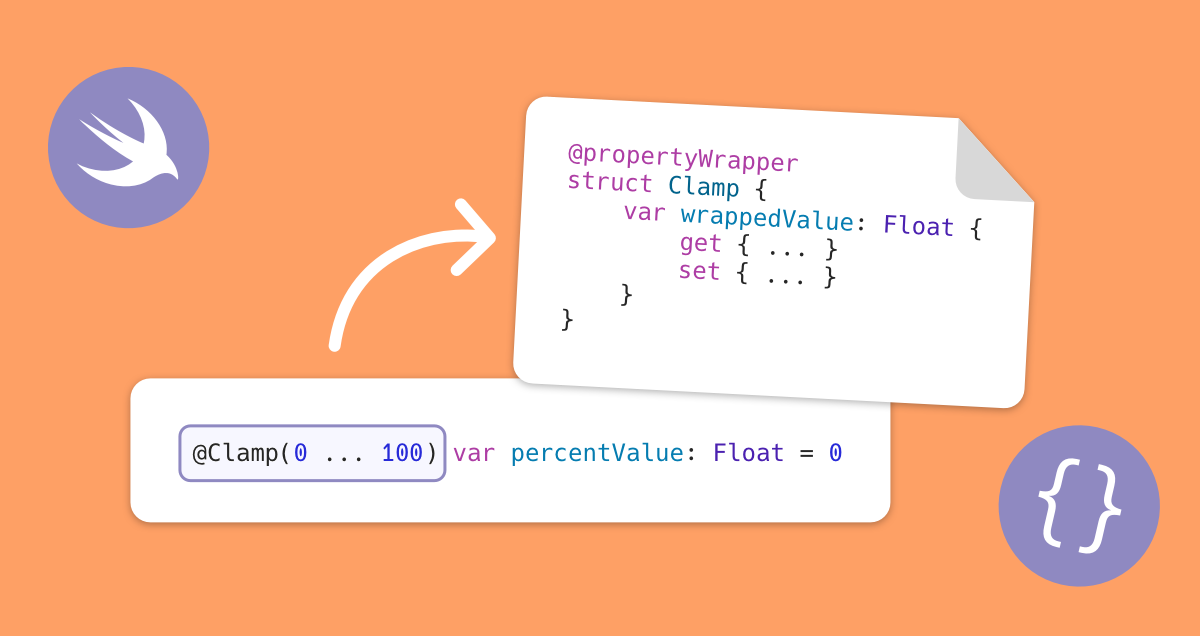# Property wrappers in Swift

by @ralfebert · updated November 20, 2021
EnglishIn SwiftUI, the use of property wrappers such as @State is a very common practice. Let's take a look behind the scenes: How exactly do property wrappers work and how can you define them on your own?## Tutorial

2. Run the unit tests in the project via Product » Test ⌘U. These check if the property percentValue stays in the value range of 0...100 (if a value smaller than 0 is set, 0 should be used instead, etc.). These will fail because the implementation is missing:3. Add a didSet block to ExampleModel and implement a check for the allowed range. Run the tests to check that the implementation works correctly.

```struct ExampleModel {
var percentValue = 5.0 {
didSet {
if self.percentValue < 0 {
self.percentValue = 0
}
else if self.percentValue > 100 {
self.percentValue = 100
}
}
}
}
```
4. With a property wrapper, this code can be extracted in a reusable form and be used for other properties.

Implement a struct and declare it as @propertyWrapper. Implement a property wrappedValue - access to the property will be delegated to this property. Move the didSet block to this property. Use the property wrapper in ExampleModel:

```@propertyWrapper
struct PercentValue {
var wrappedValue: Float {
didSet {
if self.wrappedValue < 0 {
self.wrappedValue = 0
} else if self.wrappedValue > 100 {
self.wrappedValue = 100
}
}
}
}

struct ExampleModel {
@PercentValue var percentValue: Float
}
```
5. Access to a property that has been wrapped with a property wrapper (percentValue in the example) is delegated to the wrappedValue property in the property wrapper. The compiler will internally generate the following code to implement this:

```struct ExampleModel {
var _percentValue : PercentValue

var percentValue : Float {
get {
_percentValue.wrappedValue
}
set {
_percentValue.wrappedValue = newValue
}
}
}
```

1. The logic of the property wrapper can be shortened even a bit - implement the value check with the min/max functions and check this with the unit tests.

```var wrappedValue: Float {
didSet {
self.wrappedValue = min(100, max(0, self.wrappedValue))
}
}
```
2. Extend the property wrapper to support arbitrary ranges of values. Rename the property wrapper to Clamp. Add a property range : ClosedRange<Float> and generate an initializer with Refactor » Generate Memberwise initializer (note the order of arguments: first wrappedValue, then range). Use the properties lowerBound and upperBound of the range for the implementation.

```@propertyWrapper
struct Clamp {
init(wrappedValue: Float, range: ClosedRange<Float>) {
self.wrappedValue = wrappedValue
self.range = range
}

var wrappedValue: Float {
didSet {
self.wrappedValue = min(100, max(0, self.wrappedValue))
}
}

let range: ClosedRange<Float>
}

struct ExampleModel {
@Clamp(range: 0...100) var percentValue = 5.0
}
```
3. Replace the use of the Float type with a generic argument <Value : Comparable> to support arbitrary types (they just have to be comparable, i.e. conform to the Comparable protocol).

```@propertyWrapper
struct Clamp <Value: Comparable> {
init(wrappedValue: Value, range: ClosedRange<Value>) {
self.wrappedValue = wrappedValue
self.range = range
}

var wrappedValue: Value {
didSet {
self.wrappedValue = min(range.upperBound, max(range.lowerBound, self.wrappedValue))
}
}

let range: ClosedRange<Value>
}

struct ExampleModel {
@Clamp(0...100) var percentValue: Float = 0
@Clamp(0...100) var intValue: Int = 0
}
```
4. Since Swift 5.5 / Xcode 13 property wrappers can also be used for variables and arguments. Try this in a new unit test method and implement the property wrapper so that the value range is also enforced for the initially set value.

```func testClampVariable() {
@Clamp(range: 0 ... 10) var value = -1
XCTAssertEqual(0, value)
value = 11
XCTAssertEqual(10, value)
}
```